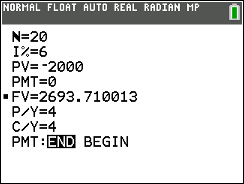# Activities

•• ##### Subject Area

• Math: Precalculus: Exponential and Logarithmic Functions

9-12

50 Minutes

• ##### Device
• TI-84 Plus Silver Edition
•TI-84 Plus C Silver Edition
•TI-84 Plus CE

## Compound Interest#### Activity Overview

This activity involves exploring the formula for compound interest as a function of the initial deposit, interest rate, and the number of pay periods per year.

#### Objectives

•  Students will interpret the variables in the formula for compound interest.
•  Students will use the formula for compound interest and understand the effects of changes in the interest rate and the number of compounding periods.
•  Students will understand the relationship between compound interest and continuous compounding

#### Vocabulary

• compound interest
• interest rate
• pay periods
• initial deposit
• continuous compounding